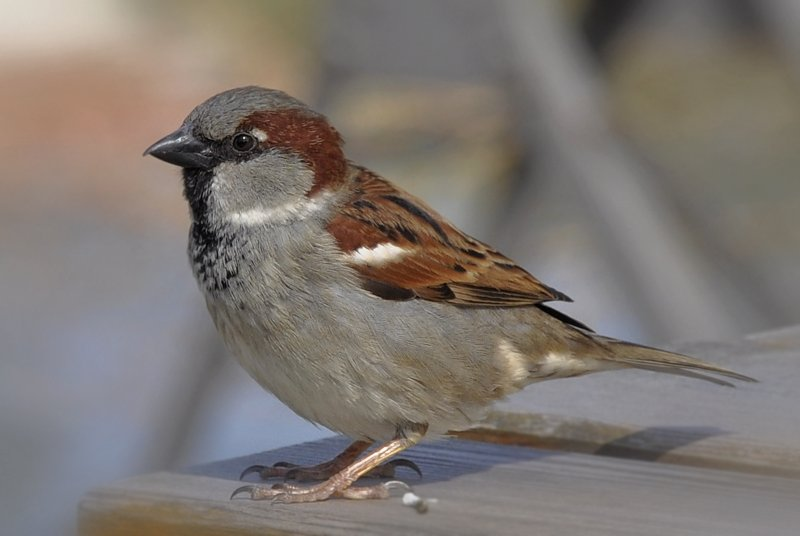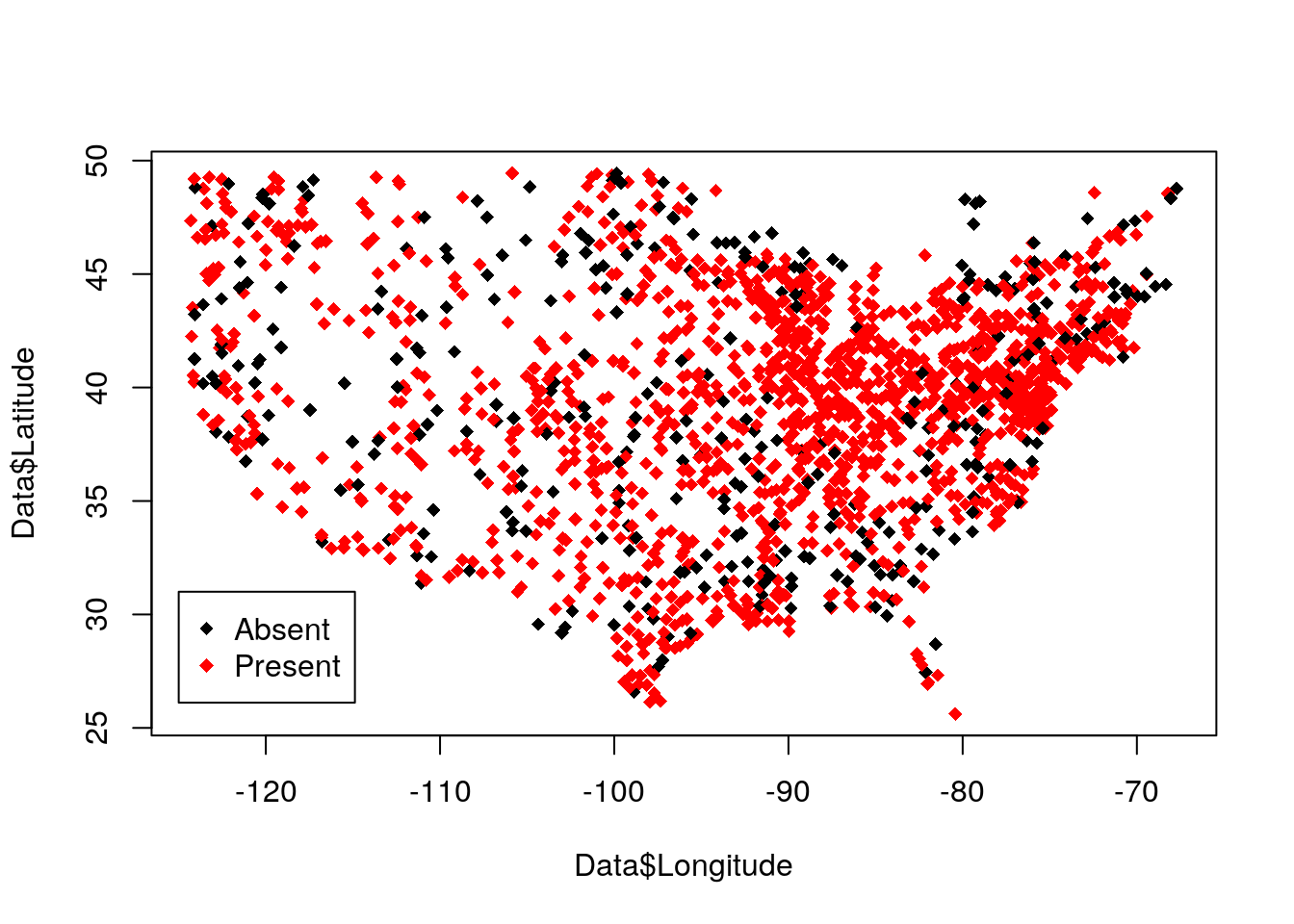## Instructions:

Hints and reminders are italic

Questions appear in blue.

Answers are in red or in R code

### Remember to read ALL questions carefully and answer ALL partsSparrow

• Photo credit Wikipedia

# More House Sparrows in North America

The North American Breeding Bird Survey is conducted across North America to estimate the abundances of birds. Bird watchers go to sites across North America and count the number of birds they observe for a set time. This data can be used to ask a wide range of questions. Here we can look at the effects of climate on abundance of the house sparrow.

The data set consists of 1714 observations at different sites across North America. For each site the presence of the house sparrow was recorded. Mean temperature (temp.mean) and total precipitation (perc.mean) were extracted from a standard database (CRU). The mean temperaure and precipitation are both scaled, so that a temperature value of 0.3 would mean that the temperature is 0.3 standard deviations above the mean in North-America.

Data <- read.csv("https://www.math.ntnu.no/emner/ST2304/2019v/Week12/HouseSparrowPresence.csv")

plot(Data$Longitude, Data$Latitude, col=1+Data\$Present, pch=18)
legend(-125, 31, c("Absent", "Present"), col=1:2, pch=18)First, fit a generalised linear model for Present assuming a binomial response, with mean temperature and total precipitation as explanatory variables. Decide which link function and model to use.

1. Which link function do you want to use? Justify your choice.

2. Write down the assumptions of this model and an equation for the expected number of sparrows at a site.

3. What is the estimated coefficient for the effect of mean temperature (prec.temp.sc)? And what is the 95% confidence interval for this coefficient? Does this suggest an effect of temperature?

4. What is the predicted probability of sparrows being found in the following sites:

1. at a site near Seattle where the mean temperature is 0.3 standard deviations below the mean, and total precipitation is 0.3 standard deviations above the mean? 2. at a site just west of Seattle, in a temperate rainforest, where the mean temperature is 0.4 standard deviations below the average, but the total precipitation is 5.8 standard deviations above the average.

From ecological theory, we might expect that there is an optimum temperature and precipitation for the sparrows, and abundance declines when the conditions are further away from the optimum. We can model this using a quadratic curve.

Fit a model with quadratic effects for temperature and precipitation.

5. Does the ecological theory seem reasonable for this data? What parameter value(s) tell you about this?

6. What is the predicted number of sparrows for the two sites mentioned above?

7. Comment briefly on the differences in the predictions from the linear and quadratic model.